GATE  >  Practice Test: Gate Civil Engineering(CE) 2012 Paper

# Practice Test: Gate Civil Engineering(CE) 2012 Paper

Test Description

## 65 Questions MCQ Test | Practice Test: Gate Civil Engineering(CE) 2012 Paper

Practice Test: Gate Civil Engineering(CE) 2012 Paper for GATE 2023 is part of GATE preparation. The Practice Test: Gate Civil Engineering(CE) 2012 Paper questions and answers have been prepared according to the GATE exam syllabus.The Practice Test: Gate Civil Engineering(CE) 2012 Paper MCQs are made for GATE 2023 Exam. Find important definitions, questions, notes, meanings, examples, exercises, MCQs and online tests for Practice Test: Gate Civil Engineering(CE) 2012 Paper below.
Solutions of Practice Test: Gate Civil Engineering(CE) 2012 Paper questions in English are available as part of our course for GATE & Practice Test: Gate Civil Engineering(CE) 2012 Paper solutions in Hindi for GATE course. Download more important topics, notes, lectures and mock test series for GATE Exam by signing up for free. Attempt Practice Test: Gate Civil Engineering(CE) 2012 Paper | 65 questions in 180 minutes | Mock test for GATE preparation | Free important questions MCQ to study for GATE Exam | Download free PDF with solutions
 1 Crore+ students have signed up on EduRev. Have you?
Practice Test: Gate Civil Engineering(CE) 2012 Paper - Question 1

### Q. 1 – Q. 5 carry one mark each. Q.1 Choose the most appropriate alternative from the options given below to complete the following sentence: Despite several ––––––––– the mission succeeded in its attempt to resolve the conflict.

Detailed Solution for Practice Test: Gate Civil Engineering(CE) 2012 Paper - Question 1

Setback means a reversal or hindrance in progress. The sentence wants to express that despite several hindrances the mission was successful in its objective.

Practice Test: Gate Civil Engineering(CE) 2012 Paper - Question 2

### The cost function for a product in a firm is given by 5q2, where q is the amount of production. The firm can sell the product at a market price of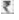50 per unit. The number of units to be produced by the firm such that the profit is maximized is

Detailed Solution for Practice Test: Gate Civil Engineering(CE) 2012 Paper - Question 2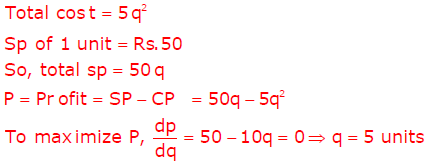Practice Test: Gate Civil Engineering(CE) 2012 Paper - Question 3

### Choose the most appropriate alternative from the options given below to complete the following sentence: Suresh’s dog is the one ––––––––– was hurt in the stampede.

Detailed Solution for Practice Test: Gate Civil Engineering(CE) 2012 Paper - Question 3

Who and whom are used for people and human beings.
That is used when the part following it cannot be avoided and the sentence will be incomplete without it.
E.g. Suresh Dog is the one that was hurt in the stampede.
Which is used for the sentence which is not necessary to include in the sentence,
E.g. Diamonds, which are expensive, often elicit forgiveness.

Practice Test: Gate Civil Engineering(CE) 2012 Paper - Question 4

Choose the grammatically INCORRECT sentence:

Detailed Solution for Practice Test: Gate Civil Engineering(CE) 2012 Paper - Question 4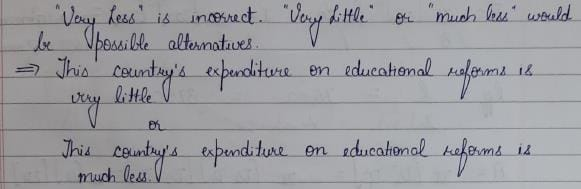Practice Test: Gate Civil Engineering(CE) 2012 Paper - Question 5

Which one of the following options is the closest in meaning to the word given below?
Mitigate

Detailed Solution for Practice Test: Gate Civil Engineering(CE) 2012 Paper - Question 5

Mitigate means making (something bad) less severe, serious or painful. Therefore, diminish is a synonym of mitigate.

Practice Test: Gate Civil Engineering(CE) 2012 Paper - Question 6

Q. 6 - Q. 10 carry two marks each.

Q.6 A political party orders an arch for the entrance to the ground in which the annual convention is being held. The profile of the arch follows the equation y = 2x – 0.1x2 where y is the height of the arch in meters. The maximum possible height of the arch is

Detailed Solution for Practice Test: Gate Civil Engineering(CE) 2012 Paper - Question 6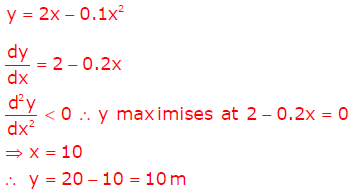Practice Test: Gate Civil Engineering(CE) 2012 Paper - Question 7

Wanted Temporary, Part-time persons for the post of Field Interviewer to conduct personal interviews to collect and collate economic data. Requirements: High School-pass, must be available for Day, Evening and Saturday work. Transportation paid, expenses reimbursed.

Which one of the following is the best inference from the above advertisement?

Detailed Solution for Practice Test: Gate Civil Engineering(CE) 2012 Paper - Question 7

There is no mention of gender in the question. Thus, one can conclude only at the time of interview whether the job is gender discriminatory or not.
Xenophobic means having or showing a dislike of or prejudice against people from other countries. However, no such statement is also made.
Thus, eliminating the options it can be concluded that the advertisement is not designed to make the post attractive.

Practice Test: Gate Civil Engineering(CE) 2012 Paper - Question 8

Given the sequence of terms, AD CG FK JP, the next term is

Detailed Solution for Practice Test: Gate Civil Engineering(CE) 2012 Paper - Question 8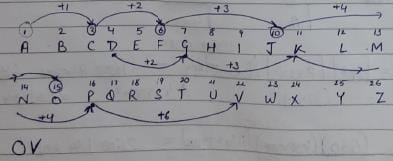Practice Test: Gate Civil Engineering(CE) 2012 Paper - Question 9

Which of the following assertions are CORRECT?
P: Adding 7 to each entry in a list adds 7 to the mean of the list
Q: Adding 7 to each entry in a list adds 7 to the standard deviation of the list
R: Doubling each entry in a list doubles the mean of the list
S: Doubling each entry in a list leaves the standard deviation of the list unchanged

Detailed Solution for Practice Test: Gate Civil Engineering(CE) 2012 Paper - Question 9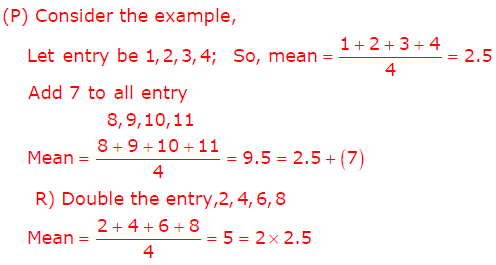Practice Test: Gate Civil Engineering(CE) 2012 Paper - Question 10

An automobile plant contracted to buy shock absorbers from two suppliers X and Y. X supplies 60% and Y supplies 40% of the shock absorbers. All shock absorbers are subjected to a quality test. The ones that pass the quality test are considered reliable. Of X’s shock absorbers, 96% are reliable. Of Y’s shock absorbers, 72% are reliable.

The probability that a randomly chosen shock absorber, which is found to be reliable, is made by Y is

Detailed Solution for Practice Test: Gate Civil Engineering(CE) 2012 Paper - Question 10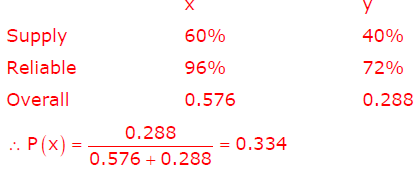Practice Test: Gate Civil Engineering(CE) 2012 Paper - Question 11

Q. 11 – Q. 35 carry one mark each.

The estimate of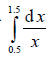obtained using Simpson’s rule with three-point function evaluation exceeds the exact value by

Detailed Solution for Practice Test: Gate Civil Engineering(CE) 2012 Paper - Question 11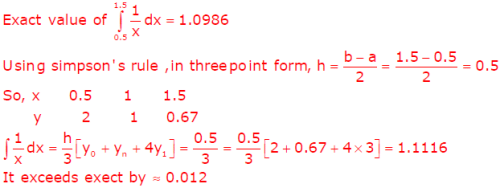Practice Test: Gate Civil Engineering(CE) 2012 Paper - Question 12

The annual precipitation data of a city is normally distributed with mean and standard deviation as 1000 mm and 200 mm, respectively. The probability that the annual precipitation will be more than 1200 mm is

Detailed Solution for Practice Test: Gate Civil Engineering(CE) 2012 Paper - Question 12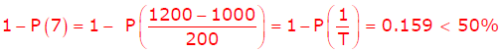Practice Test: Gate Civil Engineering(CE) 2012 Paper - Question 13

The infinite series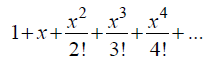corresponds to

Detailed Solution for Practice Test: Gate Civil Engineering(CE) 2012 Paper - Question 13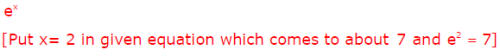Practice Test: Gate Civil Engineering(CE) 2012 Paper - Question 14

The Poisson’s ratio is defined as

Detailed Solution for Practice Test: Gate Civil Engineering(CE) 2012 Paper - Question 14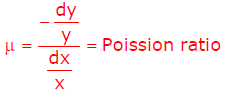Practice Test: Gate Civil Engineering(CE) 2012 Paper - Question 15

The following statements are related to bending of beams:

I The slope of the bending moment diagram is equal to the shear force.
II The slope of the shear force diagram is equal to the load intensity.
III The slope of the curvature is equal to the flexural rotation.
IV The second derivative of the deflection is equal to the curvature.

The only FALSE statement is

Detailed Solution for Practice Test: Gate Civil Engineering(CE) 2012 Paper - Question 15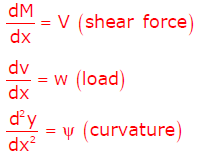Practice Test: Gate Civil Engineering(CE) 2012 Paper - Question 16

If a small concrete cube is submerged deep in still water in such a way that the pressure exerted on all faces of the cube is p, then the maximum shear stress developed inside the cube is

Detailed Solution for Practice Test: Gate Civil Engineering(CE) 2012 Paper - Question 16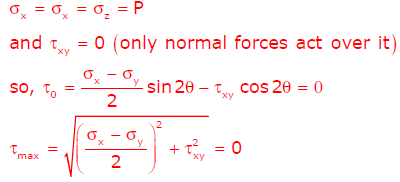Practice Test: Gate Civil Engineering(CE) 2012 Paper - Question 17

As per IS 456:2000, in the Limit State Design of a flexural member, the strain in reinforcing bars under tension at ultimate state should not be less than

Detailed Solution for Practice Test: Gate Civil Engineering(CE) 2012 Paper - Question 17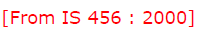Practice Test: Gate Civil Engineering(CE) 2012 Paper - Question 18

Which one of the following is categorised as a long-term loss of prestress in a prestressed concrete member?

Detailed Solution for Practice Test: Gate Civil Engineering(CE) 2012 Paper - Question 18

 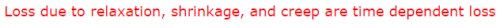Practice Test: Gate Civil Engineering(CE) 2012 Paper - Question 19

In a steel plate with bolted connections, the rupture of the net section is a mode of failure under

Detailed Solution for Practice Test: Gate Civil Engineering(CE) 2012 Paper - Question 19

Steel plate with bolted section may fail in following three cases-
A. Gross yielding of section
B. Net Tension Rupture if the Section
C. Block Shear Failure

Practice Test: Gate Civil Engineering(CE) 2012 Paper - Question 20

The ratio of the theoretical critical buckling load for a column with fixed ends to that of another column with the same dimensions and material, but with pinned ends, is equal to

Detailed Solution for Practice Test: Gate Civil Engineering(CE) 2012 Paper - Question 20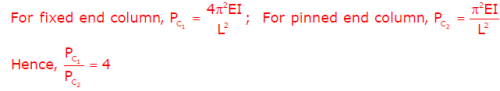Practice Test: Gate Civil Engineering(CE) 2012 Paper - Question 21

The effective stress friction angle of a saturated, cohesionless soil is 38°. The ratio of shear stress to normal effective stress on the failure plane is

Detailed Solution for Practice Test: Gate Civil Engineering(CE) 2012 Paper - Question 21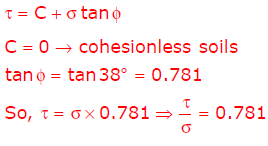Practice Test: Gate Civil Engineering(CE) 2012 Paper - Question 22

Two series of compaction tests were performed in the laboratory on an inorganic clayey soil
employing two different levels of compaction energy per unit volume of soil. With regard to the
above tests, the following two statements are made.

I The optimum moisture content is expected to be more for the tests with higher energy.
II The maximum dry density is expected to be more for the tests with higher energy.

The CORRECT option evaluating the above statements is

Detailed Solution for Practice Test: Gate Civil Engineering(CE) 2012 Paper - Question 22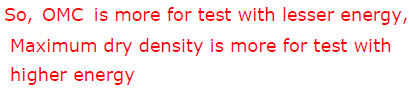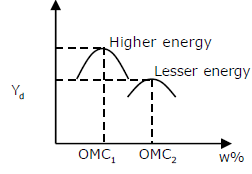Practice Test: Gate Civil Engineering(CE) 2012 Paper - Question 23

As per the Indian Standard soil classification system, a sample of silty clay with liquid limit of 40% and plasticity index of 28% is classified as

Detailed Solution for Practice Test: Gate Civil Engineering(CE) 2012 Paper - Question 23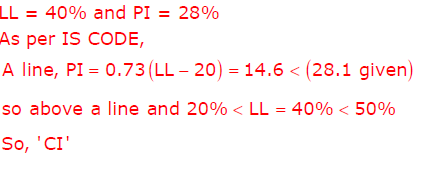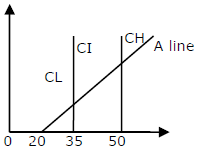Practice Test: Gate Civil Engineering(CE) 2012 Paper - Question 24

A smooth rigid retaining wall moves as shown in the sketch causing the backfill material to fail. The backfill material is homogeneous and isotropic, and obeys the Mohr-Coulomb failure criterion. The major principal stress is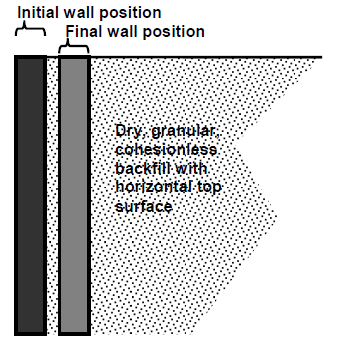Detailed Solution for Practice Test: Gate Civil Engineering(CE) 2012 Paper - Question 24

The question provides all the assumptions of Rankine Theory’s of earth pressure in the statement like smooth wall surface, dry cohesionless sand, vertical retaining wall.
Moreover, it is the case of horizontal backfill without any surcharge. As the wall move towards the soil thus, it will lead to the development of passive earth pressure acting normal to the wall face.

Practice Test: Gate Civil Engineering(CE) 2012 Paper - Question 25

An embankment is to be constructed with a granular soil (bulk unit weight = 20 kN/m3) on a saturated clayey silt deposit (undrained shear strength = 25 kPa). Assuming undrained general shear failure and bearing capacity factor of 5.7, the maximum height (in m) of the embankment at the point of failure is

Practice Test: Gate Civil Engineering(CE) 2012 Paper - Question 26

A trapezoidal channel is 10.0 m wide at the base and has a side slope of 4 horizontal to 3 vertical. The bed slope is 0.002. The channel is lined with smooth concrete (Manning’s n = 0.012). The hydraulic radius (in m) for a depth of flow of 3.0 m is

Detailed Solution for Practice Test: Gate Civil Engineering(CE) 2012 Paper - Question 26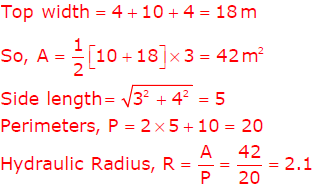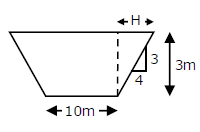Practice Test: Gate Civil Engineering(CE) 2012 Paper - Question 27

A rectangular open channel of width 5.0 m is carrying a discharge of 100 m3/s. The Froude number of the flow is 0.8. The depth of flow (in m) in the channel is

Detailed Solution for Practice Test: Gate Civil Engineering(CE) 2012 Paper - Question 27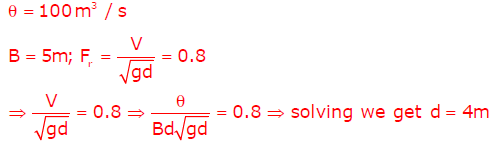Practice Test: Gate Civil Engineering(CE) 2012 Paper - Question 28

The circular water pipes shown in the sketch are flowing full. The velocity of flow (in m/s) in the branch pipe “R” is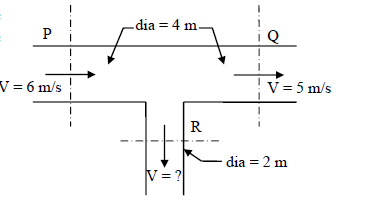Detailed Solution for Practice Test: Gate Civil Engineering(CE) 2012 Paper - Question 28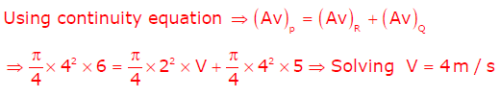Practice Test: Gate Civil Engineering(CE) 2012 Paper - Question 29

The ratio of actual evapo-transpiration to potential evapo-transpiration is in the range

Detailed Solution for Practice Test: Gate Civil Engineering(CE) 2012 Paper - Question 29

Potential Evapotranspiration is the maximum possible evapotranspiration had there been no shortage of water.
Actual Evapotranspiration is the actual evapotranspiration taking place in present moisture conditions.
Actual Evapotranspiration <= Potential Evapotranspiration
Thus, ratio varies from 0 to 1.

Practice Test: Gate Civil Engineering(CE) 2012 Paper - Question 30

A sample of domestic sewage is digested with silver sulphate, sulphuric acid, potassium dichromate and mercuric sulphate in chemical oxygen demand (COD) test. The digested sample is then titrated with standard ferrous ammonium sulphate (FAS) to determine the un-reacted amount of

Practice Test: Gate Civil Engineering(CE) 2012 Paper - Question 31

Assertion [a]: At a manhole, the crown of the outgoing sewer should not be higher than the crown of the incoming sewer.
Reason [r]: Transition from a larger diameter incoming sewer to a smaller diameter outgoing sewer at a manhole should not be made.

The CORRECT option evaluating the above statements is :

Detailed Solution for Practice Test: Gate Civil Engineering(CE) 2012 Paper - Question 31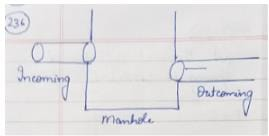The outgoing sewer should always be lower than the incoming sewer for free flow (nor the sewer will flow back to the incoming sewer pipe).
The diameter of the outgoing sewer should be equal to or greater than the diameter of incoming sewer to avoid any clogging situation..
Thus, both the statements are correct but the reason does not explain the statement.

Practice Test: Gate Civil Engineering(CE) 2012 Paper - Question 32

Two major roads with two lanes each are crossing in an urban area to form an un-controlled intersection. The number of conflict points when both roads are one-way is “X” and when both roads are two-way is “Y”. The ratio of X to Y is

Detailed Solution for Practice Test: Gate Civil Engineering(CE) 2012 Paper - Question 32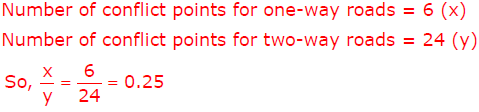Practice Test: Gate Civil Engineering(CE) 2012 Paper - Question 33

Two bitumen samples “X” and “Y” have softening points 45°C and 60°C, respectively. Consider the following statements:

I. I Viscosity of “X” will be higher than that of “Y” at the same temperature.
II Penetration value of “X” will be lesser than that of “Y” under standard conditions.

The CORRECT option evaluating the above statements is

Detailed Solution for Practice Test: Gate Civil Engineering(CE) 2012 Paper - Question 33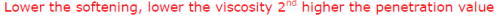Practice Test: Gate Civil Engineering(CE) 2012 Paper - Question 34

Detailed Solution for Practice Test: Gate Civil Engineering(CE) 2012 Paper - Question 34

The instrument can be used for direct roughness measurement or for calibrating other equipment like Bump Integrator. In RHD Merlin is used for calibrating purpose. It consists of a metal frame 1.8 meters long with a wheel at the front, a foot at the rear and a probe midway between them which rests on the road surface.

Practice Test: Gate Civil Engineering(CE) 2012 Paper - Question 35

Which of the following errors can be eliminated by reciprocal measurements in differential leveling?

I Error due to earth’s curvature
II Error due to atmospheric refraction

Detailed Solution for Practice Test: Gate Civil Engineering(CE) 2012 Paper - Question 35

Reciprocal Levelling involves keeping the instrument at A and taking reading of B and vice versa. Thus, the method eliminates any possibility of error due to the inclined line of collimation, earth’s curvature and atmospheric refraction.

Practice Test: Gate Civil Engineering(CE) 2012 Paper - Question 36

Q. 36 - Q. 65 carry two marks each.

The error in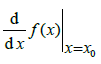for a continuous function estimated with h = 0.03 using the central difference formula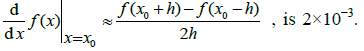The values of x0 and f(x0) are 19.78 and 500.01, respectively. The corresponding error in the central difference estimate for h = 0.02 is approximately

Practice Test: Gate Civil Engineering(CE) 2012 Paper - Question 37

In an experiment, positive and negative values are equally likely to occur. The probability of obtaining at most one negative value in five trials is

Detailed Solution for Practice Test: Gate Civil Engineering(CE) 2012 Paper - Question 37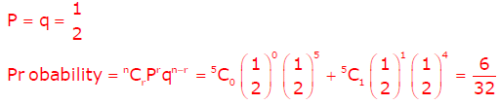Practice Test: Gate Civil Engineering(CE) 2012 Paper - Question 38

The eigenvalues of matrix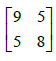are

Detailed Solution for Practice Test: Gate Civil Engineering(CE) 2012 Paper - Question 38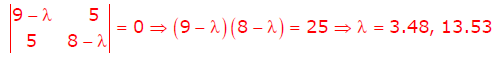Practice Test: Gate Civil Engineering(CE) 2012 Paper - Question 39

For the parallelogram OPQR shown in the sketch,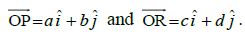The area of the parallelogram is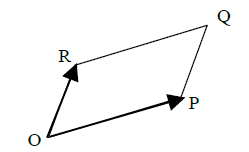Detailed Solution for Practice Test: Gate Civil Engineering(CE) 2012 Paper - Question 39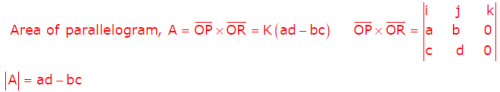Practice Test: Gate Civil Engineering(CE) 2012 Paper - Question 40

The solution of the ordinary differential equation dy/dx+2y=0 for the boundary condition, y = 5 at x = 1 is

Detailed Solution for Practice Test: Gate Civil Engineering(CE) 2012 Paper - Question 40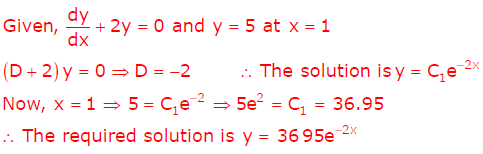Practice Test: Gate Civil Engineering(CE) 2012 Paper - Question 41

A simply supported beam is subjected to a uniformly distributed load of intensity w per unit length, on half of the span from one end. The length of the span and the flexural stiffness are denoted as l and EI, respectively. The deflection at mid-span of the beam is

Detailed Solution for Practice Test: Gate Civil Engineering(CE) 2012 Paper - Question 41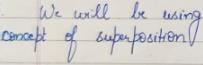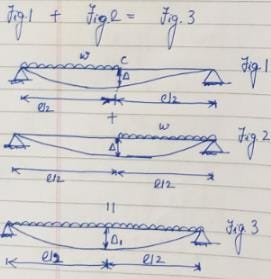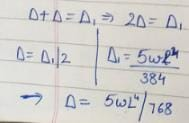Practice Test: Gate Civil Engineering(CE) 2012 Paper - Question 42

The sketch shows a column with a pin at the base and rollers at the top. It is subjected to an axial force P and a moment M at mid-height. The reaction(s) at R is/are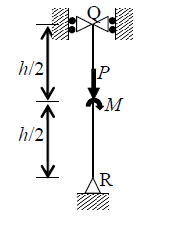Detailed Solution for Practice Test: Gate Civil Engineering(CE) 2012 Paper - Question 42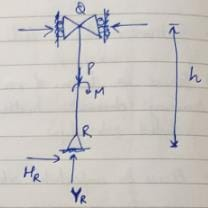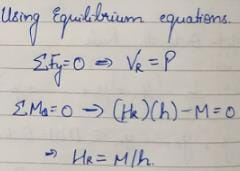Practice Test: Gate Civil Engineering(CE) 2012 Paper - Question 43

A concrete beam prestressed with a parabolic tendon is shown in the sketch. The eccentricity of the tendon is measured from the centroid of the cross-section. The applied prestressing force at service is 1620 kN. The uniformly distributed load of 45 kN/m includes the self-weight.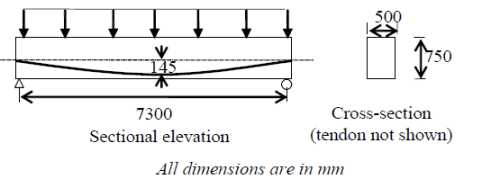The stress (in N/mm2) in the bottom fibre at mid-span is

Detailed Solution for Practice Test: Gate Civil Engineering(CE) 2012 Paper - Question 43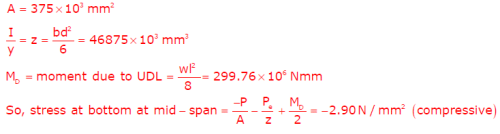Practice Test: Gate Civil Engineering(CE) 2012 Paper - Question 44

A symmetric frame PQR consists of two inclined members PQ and QR, connected at ‘Q’ with a rigid joint, and hinged at ‘P’ and ‘R’. The horizontal length PR is l. If a weight W is suspended at ‘Q’, the bending moment at ‘Q’ is

Detailed Solution for Practice Test: Gate Civil Engineering(CE) 2012 Paper - Question 44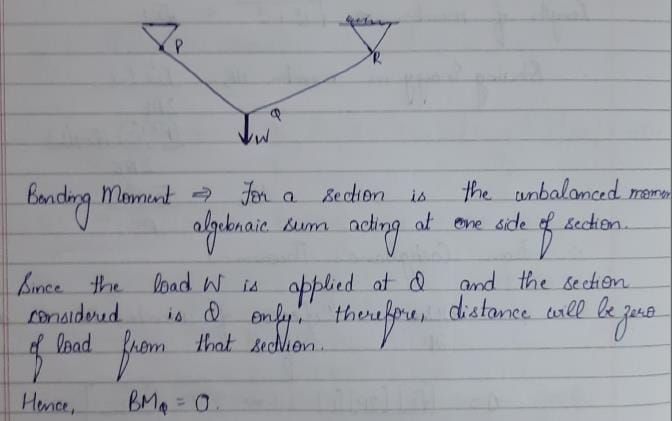Practice Test: Gate Civil Engineering(CE) 2012 Paper - Question 45

Two plates are connected by fillet welds of size 10 mm and subjected to tension, as shown in the sketch. The thickness of each plate is 12 mm. The yield stress and the ultimate tensile stress of steel are 250 MPa and 410 MPa, respectively. The welding is done in the workshop (λmw = 1.25). As per the Limit State Method of IS 800:2007, the minimum length (rounded off to the nearest higher multiple of 5 mm) of each weld to transmit a force P equal to 270 kN is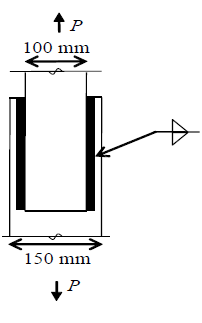Practice Test: Gate Civil Engineering(CE) 2012 Paper - Question 46

Two soil specimens with identical geometric dimensions were subjected to falling head permeability tests in the laboratory under identical conditions. The fall of water head was measured after an identical time interval. The ratio of initial to final water heads for the test involving the first specimen was 1.25. If the coefficient of permeability of the second specimen is 5-times that of the first, the ratio of initial to final water heads in the test involving the second specimen is

Detailed Solution for Practice Test: Gate Civil Engineering(CE) 2012 Paper - Question 46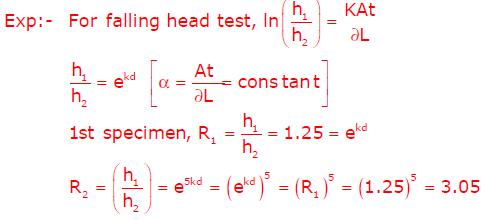Practice Test: Gate Civil Engineering(CE) 2012 Paper - Question 47

A layer of normally consolidated, saturated silty clay of 1 m thickness is subjected to one dimensional consolidation under a pressure increment of 20 kPa. The properties of the soil are: specific gravity = 2.7, natural moisture content = 45%, compression index = 0.45, and recompression index = 0.05. The initial average effective stress within the layer is 100 kPa. Assuming Terzaghi’s theory to be applicable, the primary consolidation settlement (rounded off to the nearest mm) is

Detailed Solution for Practice Test: Gate Civil Engineering(CE) 2012 Paper - Question 47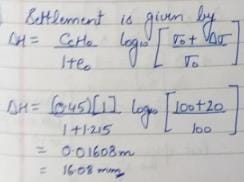Practice Test: Gate Civil Engineering(CE) 2012 Paper - Question 48

Steady state seepage is taking place through a soil element at Q, 2 m below the ground surface immediately downstream of the toe of an earthen dam as shown in the sketch. The water level in a piezometer installed at P, 500 mm above Q, is at the ground surface. The water level in a piezometer installed at R, 500 mm below Q, is 100 mm above the ground surface. The bulk saturated unit weight of the soil is 18 kN/m3 and the unit weight of water is 9.81 kN/m3. The vertical effective stress (in kPa) at Q is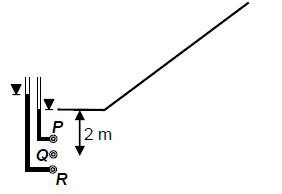Detailed Solution for Practice Test: Gate Civil Engineering(CE) 2012 Paper - Question 48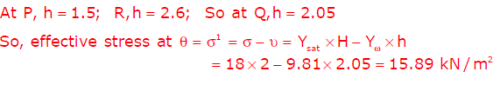Practice Test: Gate Civil Engineering(CE) 2012 Paper - Question 49

The top width and the depth of flow in a triangular channel were measured as 4 m and 1 m, respectively. The measured velocities on the centre line at the water surface, 0.2 m and 0.8 m below the surface are 0.7 m/s, 0.6 m/s and 0.4 m/s, respectively. Using two-point method of velocity measurement, the discharge (in m3/s) in the channel is

Detailed Solution for Practice Test: Gate Civil Engineering(CE) 2012 Paper - Question 49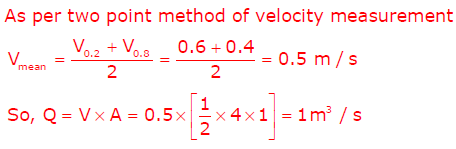Practice Test: Gate Civil Engineering(CE) 2012 Paper - Question 50

A water treatment plant is required to process 28800 m3/d of raw water (density = 1000 kg/m3, kinematic viscosity = 10-6 m2/s). The rapid mixing tank imparts tank a velocity gradient of 900s-1 to blend 35 mg/l of alum with the flow for a detention time of 2 minutes. The power input (W) required for rapid mixing is

Practice Test: Gate Civil Engineering(CE) 2012 Paper - Question 51

Wheat crop requires 55 cm of water during 120 days of base period. The total rainfall during this period is 100 mm. Assume the irrigation efficiency to be 60%. The area (in ha) of the land which can be irrigated with a canal flow of 0.01 m3/s is

Practice Test: Gate Civil Engineering(CE) 2012 Paper - Question 52

A water sample has a pH of 9.25. The concentration of hydroxyl ions in the water sample is

Detailed Solution for Practice Test: Gate Civil Engineering(CE) 2012 Paper - Question 52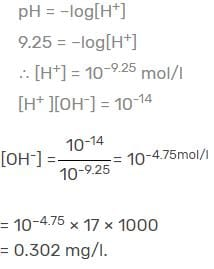Practice Test: Gate Civil Engineering(CE) 2012 Paper - Question 53

A town is required to treat 4.2 m3/min of raw water for daily domestic supply. Flocculating particles are to be produced by chemical coagulation. A column analysis indicated that an overflow rate of 0.2 mm/s will produce satisfactory particle removal in a settling basin at a depth of 3.5 m. The required surface area (in m2 ) for settling is

Detailed Solution for Practice Test: Gate Civil Engineering(CE) 2012 Paper - Question 53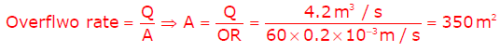Practice Test: Gate Civil Engineering(CE) 2012 Paper - Question 54

A pavement designer has arrived at a design traffic of 100 million standard axles for a newly developing national highway as per IRC:37 guidelines using the following data: design life = 15 years, commercial vehicle count before pavement construction = 4500 vehicles/day, annual traffic growth rate = 8%. The vehicle damage factor used in the calculation was

Detailed Solution for Practice Test: Gate Civil Engineering(CE) 2012 Paper - Question 54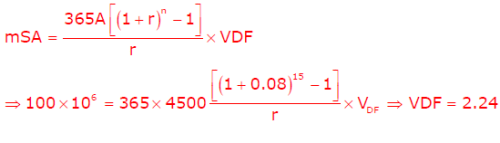Practice Test: Gate Civil Engineering(CE) 2012 Paper - Question 55

The following data are related to a horizontal curved portion of a two-lane highway: length of curve = 200 m, radius of curve = 300 m and width of pavement = 7.5 m. In order to provide a stopping sight distance (SSD) of 80 m, the set back distance (in m) required from the centre line of the inner lane of the pavement is

Practice Test: Gate Civil Engineering(CE) 2012 Paper - Question 56

A two-lane urban road with one-way traffic has a maximum capacity of 1800 vehicles/hour. Under the jam condition, the average length occupied by the vehicles is 5.0 m. The speed versus density relationship is linear. For a traffic volume of 1000 vehicles/hour, the density (in vehicles/km) is

Practice Test: Gate Civil Engineering(CE) 2012 Paper - Question 57

The horizontal distance between two stations P and Q is 100 m. The vertical angles from P and Q to the top of a vertical tower at T are 3° and 5° above horizontal, respectively. The vertical angles from P and Q to the base of the tower are 0.1° and 0.5° below horizontal, respectively. Stations P, Q and the tower are in the same vertical plane with P and Q being on the same side of T. Neglecting earth’s curvature and atmospheric refraction, the height (in m) of the tower is

Detailed Solution for Practice Test: Gate Civil Engineering(CE) 2012 Paper - Question 57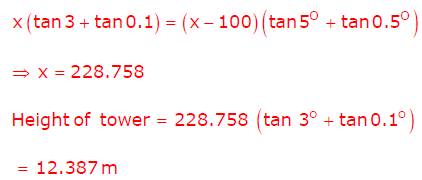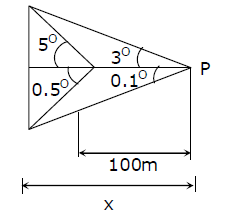Practice Test: Gate Civil Engineering(CE) 2012 Paper - Question 58

Common Data Questions
Common Data for Questions 58 and 59:

The flow net around a sheet pile wall is shown in the sketch. The properties of the soil are: permeability coefficient = 0.09 m/day (isotropic), specific gravity = 2.70 and void ratio = 0.85. The sheet pile wall and the bottom of the soil are impermeable.

The seepage loss (in m3 per day per unit length of the wall) of water is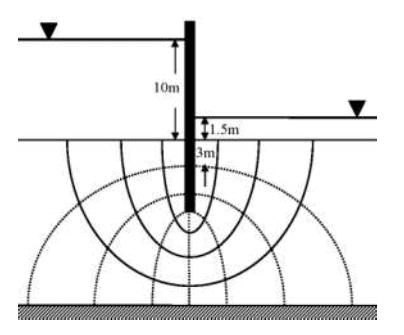Practice Test: Gate Civil Engineering(CE) 2012 Paper - Question 59

The flow net around a sheet pile wall is shown in the sketch. The properties of the soil are: permeability coefficient = 0.09 m/day (isotropic), specific gravity = 2.70 and void ratio = 0.85. The sheet pile wall and the bottom of the soil are impermeable.

The factor of safety against the occurrence of piping failure isPractice Test: Gate Civil Engineering(CE) 2012 Paper - Question 60

Common Data for Questions 60 and 61:
An activated sludge system (sketched below) is operating at equilibrium with the following information. Wastewater related data: flow rate = 500 m3/hour, influent BOD = 150 mg/L, effluent BOD = 10 mg/L. Aeration tank related data: hydraulic retention time = 8 hours, mean-cell-residence time = 240 hours, volume = 4000 m3, mixed liquor suspended solids = 2000 mg/L.

The food-to-biomass (F/M) ratio (in kg BOD per kg biomass per day) for the aeration tank is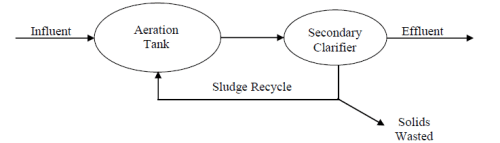Detailed Solution for Practice Test: Gate Civil Engineering(CE) 2012 Paper - Question 60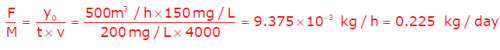Practice Test: Gate Civil Engineering(CE) 2012 Paper - Question 61

An activated sludge system (sketched below) is operating at equilibrium with the following information. Wastewater related data: flow rate = 500 m3/hour, influent BOD = 150 mg/L, effluent BOD = 10 mg/L. Aeration tank related data: hydraulic retention time = 8 hours, mean-cell-residence time = 240 hours, volume = 4000 m3, mixed liquor suspended solids = 2000 mg/L.

The mass (in kg/day) of solids wasted from the system isDetailed Solution for Practice Test: Gate Civil Engineering(CE) 2012 Paper - Question 61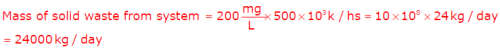Practice Test: Gate Civil Engineering(CE) 2012 Paper - Question 62

The cross-section at mid-span of a beam at the edge of a slab is shown in the sketch. A portion of the slab is considered as the effective flange width for the beam. The grades of concrete and reinforcing steel are M25 and Fe415, respectively. The total area of reinforcing bars (As) is 4000 mm2. At the ultimate limit state, xu denotes the depth of the neutral axis from the top fibre. Treat the section as under-reinforced and flanged (xu > 100 mm).

The value of xu (in mm) computed as per the Limit State Method of IS 456:2000 is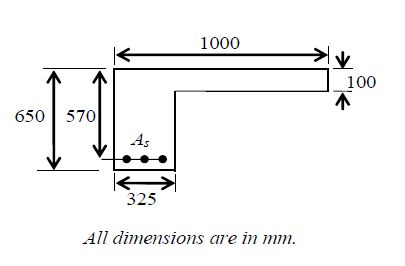Detailed Solution for Practice Test: Gate Civil Engineering(CE) 2012 Paper - Question 62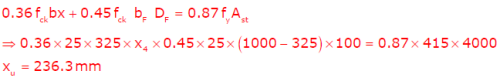Practice Test: Gate Civil Engineering(CE) 2012 Paper - Question 63

The cross-section at mid-span of a beam at the edge of a slab is shown in the sketch. A portion of the slab is considered as the effective flange width for the beam. The grades of concrete and reinforcing steel are M25 and Fe415, respectively. The total area of reinforcing bars (As) is 4000 mm2. At the ultimate limit state, xu denotes the depth of the neutral axis from the top fibre. Treat the section as under-reinforced and flanged (xu > 100 mm).

The ultimate moment capacity (in kNm) of the section, as per the Limit State Method of IS 456:2000 isPractice Test: Gate Civil Engineering(CE) 2012 Paper - Question 64

The drainage area of a watershed is 50 km2. The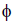index is 0.5 cm/hour and the base flow at the outlet is 10 m3/s. One hour unit hydrograph (unit depth = 1 cm) of the watershed is triangular in shape with a time base of 15 hours. The peak ordinate occurs at 5 hours.

The peak ordinate (in m3/s/cm) of the unit hydrograph is

Detailed Solution for Practice Test: Gate Civil Engineering(CE) 2012 Paper - Question 64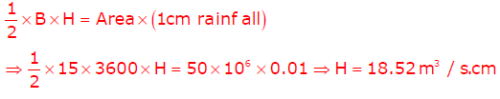Practice Test: Gate Civil Engineering(CE) 2012 Paper - Question 65

The drainage area of a watershed is 50 km2. Theindex is 0.5 cm/hour and the base flow at the outlet is 10 m3/s. One hour unit hydrograph (unit depth = 1 cm) of the watershed is triangular in shape with a time base of 15 hours. The peak ordinate occurs at 5 hours.

For a storm of depth of 5.5 cm and duration of 1 hour, the peak ordinate (in m3/s) of the hydrograph is

Detailed Solution for Practice Test: Gate Civil Engineering(CE) 2012 Paper - Question 65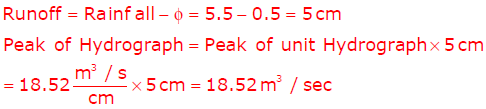Information about Practice Test: Gate Civil Engineering(CE) 2012 Paper Page
In this test you can find the Exam questions for Practice Test: Gate Civil Engineering(CE) 2012 Paper solved & explained in the simplest way possible. Besides giving Questions and answers for Practice Test: Gate Civil Engineering(CE) 2012 Paper, EduRev gives you an ample number of Online tests for practice(Scan QR code)